# ISEE Upper Level Quantitative : Squares

## Example Questions

← Previous 1 3 4

### Example Question #1 : Squares

Which is the greater quantity?

(a) The perimeter of a square with sidelength 1 meter

(b) The perimeter of a regular pentagon with sidelength 75 centimeters

(a) and (b) are equal.

(b) is greater.

It is impossible to tell from the information given.

(a) is greater.

(a) is greater.

Explanation:

(a) One meter is equal to 100 centimeters; a square with this sidelength has perimeter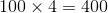centimeters.

(b) A regular pentagon has five congruent sides; since the sidelength is 75 centimeters, the perimeter is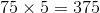centimeters.

This makes (a) greater.

### Example Question #201 : Geometry

Square 1 is inscribed inside a circle. The circle is inscribed inside Square 2.

Which is the greater quantity?

(a) Twice the perimeter of Square 1

(b) The perimeter of Square 2

(b) is greater.

(a) is greater.

It is impossible to tell from the information given.

(a) and (b) are equal.

(a) is greater.

Explanation:

Letbe the sidelength of Square 1. Then the length of a diagonal of this square - which istimes this sidelength, or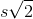by the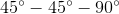Theorem - is the same as the diameter of this circle, which, in turn, is equal to the sidelength of Square 2.

Since the perimeter of a square is four times its sidelength, Square 1 has perimeter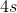; Square 2 has perimeter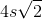, which istimes the perimeter of Square 1.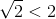, making the perimeter of Square 2 less than twice than the perimeter of Square 1.

### Example Question #3 : Squares

Five squares have sidelengths one foot, two feet, three feet, four feet, and five feet.

Which is the greater quantity?

(A) The mean of their perimeters

(B) The median of their perimeters

It is impossible to tell which is greater from the information given

(A) and (B) are equal

(B) is greater

(A) is greater

(A) and (B) are equal

Explanation:

The perimeters of the squares are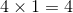feet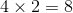feet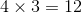feet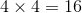feet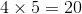feet

The mean of the perimeters is their sum divided by five;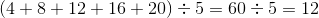feet.

The median of the perimeters is the value in the middle when they are arranged in ascending order; this can be seen to also be 12 feet.

The quantities are equal.

### Example Question #202 : Geometry

Four squares have sidelengths one meter, one meter, 120 centimeters, and 140 centimeters. Which is the greater quantity?

(A) The mean of their perimeters

(B) The median of their perimeters

(A) and (B) are equal

It is impossible to tell which is greater from the information given

(A) is greater

(B) is greater

(A) is greater

Explanation:

First find the perimeters of the squares: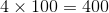centimeters (one meter being 100 centimeters)centimeters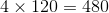centimeters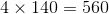centimeters

The mean of the perimeters is their sum divided by four: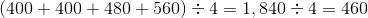feet.

The median of the perimeters is the mean of the two values in the middle, assuming the values are in numerical order: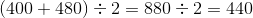The mean, (A), is greater.

### Example Question #5 : Squares

The area of a square is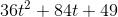.

Give the perimeter of the square.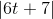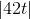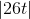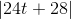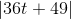Explanation:

The length of one side of a square is the square root of its area. The polynomial representing the area of the square can be recognized as a perfect square trinomial: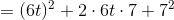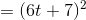Therefore, the square root of the area is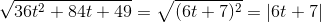,

which is the length of one side.

The perimeter of the square is four times this length, or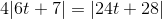.

### Example Question #6 : Squares

The perimeters of six squares form an arithmetic sequence. The second-smallest square has sides that are two inches longer than those of the smallest square.

Which, if either, is the greater quantity?

(a) The perimeter of the third-smallest square

(b) The length of one side of the largest square

(a) is greater

(b) is greater

(a) and (b) are equal

It is impossible to tell which is greater from the information given

(a) is greater

Explanation:

Let the length of one side of the first square be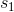. Then the length of one side of the second-smallest square is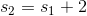, and the perimeters of the squares are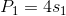and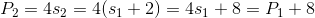This makes the common difference of the perimeters 8 units.

The perimeters of the squares being in arithmetic progression, the perimeter of theth-smallest square is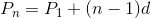Since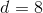, this becomes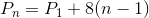The perimeter of the third-smallest square is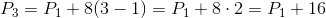The perimeter of the largest, or sixth-smallest, square is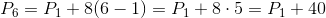The length of one side of this square is one fourth of this, or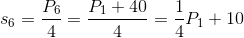Therefore, we are comparing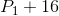and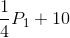.

Since a perimeter must be positive,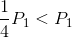;

also,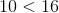.

Therefore, regardless of the value of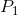,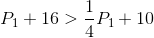,

and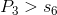,

making (a) the greater quantity.

### Example Question #11 : Quadrilaterals

The sidelength of a square is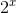. Give its perimeter in terms of.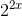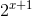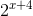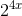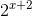Explanation:

The perimeter of a square is four times the length of a side, which here is: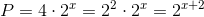### Example Question #8 : Squares

A diagonal of a square has length. Give its perimeter.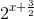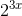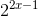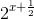Explanation:

The length of a side of a square can be determined by dividing the length of a diagonal by- that is,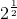. A diagonal has length, so the sidelength is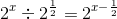Multiply this by four to get the perimeter: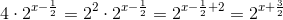### Example Question #12 : Quadrilaterals

The perimeters of six squares form an arithmetic sequence. The smallest square has area 9; the second smallest square has area 25. Give the perimeter of the largest of the six squares.

None of the other responses gives the correct answer.Explanation:

The two smallest squares have areas 9 and 25, so their sidelengths are the square roots of these, or, respectively, 3 and 5. Their perimeters are the sidelengths multiplied by four, or, respectively, 12 and 20. Therefore, in the arithmetic sequence,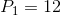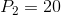and the common difference is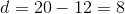.

The perimeter of theth smallest square isSetting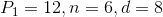, the perimeter of the largest (or sixth-smallest) square is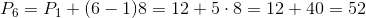.

### Example Question #10 : Squares

The perimeter of a square is one yard. Which is the greater quantity?

(a) The area of the square

(b)square foot

(a) and (b) are equal.

It is impossible to tell form the information given.

(a) is greater.

(b) is greater.

One yard is equal to three feet, so the length of one side of a square with this perimeter isfeet. The area of the square is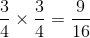square feet.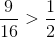, making (a) greater.